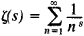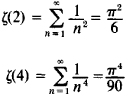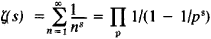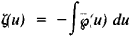# Zeta Function

Also found in: Wikipedia.

## zeta function

[′zād·ə ‚fəŋk·shən]
(mathematics)
McGraw-Hill Dictionary of Scientific & Technical Terms, 6E, Copyright © 2003 by The McGraw-Hill Companies, Inc.
The following article is from The Great Soviet Encyclopedia (1979). It might be outdated or ideologically biased.

## Zeta Function

(1) An analytic function of a complex variable s = σ + it, defined for σ > 1 by the formulaL. Euler in 1737 introduced this function in mathematical analysis for real s, and in 1859 the German mathematician G. F. B. Riemann first studied it for complex 5, and therefore it is often called the Riemann zeta function. After the works of Euler (1748, 1749), P. L. Chebyshev (1848), and Riemann the profound relation between the properties of the zeta function and the properties of prime numbers was elucidated.

Euler calculated the values of ζ(2s) for any natural s. In particularFurther, he derived the identity (the Euler identity)where the product applies to all primes p = 2, 3, 5, . . . .

The zero distribution of the zeta function is of primary importance to the theory of primes. It is known that the zeta function has zeros at the points s = -2n, where n = 1, 2, . . . (these zeros are commonly called trivial zeros) and that all other (so-called nontrivial) zeros of the zeta function lie in the strip 0 < σ < 1, which is called the critical strip. Riemann proposed that all nontrivial zeros of the zeta function lie on the straight line σ = ½ . To date this hypothesis has been neither proven nor disproven. Important results on the zero distribution of the zeta function have been obtained by a new method in analytic number theory developed by the Soviet mathematician I. M. Vinogradov.

### REFERENCES

Euler, L. Vvedenie v analiz beskonechnykh. 2nd ed., vol. 1. Moscow, 1961. (Translated from Latin.)
Wittacker, E. T., and G. Watson. Kurs sovremennogo analiza, 2nd ed., part 2. Moscow, 1963. (Translated from English.)
Titchmarsh, E. C. Dzeta-funktsiia Rimana. Moscow, 1947. (Translated from English.)
Ingham, A. E.Raspredelenie prostykh chisel Moscow-Leningrad, 1936. (Translated from English.)
Jahnke, E., and F. Emde. Tablitsy funktsii s formulami i krivymi. Moscow-Leningrad, 1948. (Translated from German.)
Prachar, K. Raspredelenie prostykh chisel. Moscow, 1967. (Translated from German.)
(2) In the theory of elliptic functions Weierstrass’ zeta function is encountered:where ζ(u) is Weierstrass’ elliptic function. This zeta function should not be confused with the Riemann zeta function.
References in periodicals archive ?
In case K = Q, the Laurent expansion of the Riemann zeta function [zeta](s) at its pole s = 1 is given by
We also use the quasi-quadratic residue codes defined below to construct an example of a formally self-dual optimal code whose zeta function does not satisfy the "Riemann hypothesis."
They cover fractional thoughts; uniqueness for weak solutions of parabolic equations with a fractional time derivative; boundary regularity for the free boundary in the one-phase problem; fractional Laplacians on the sphere, the Minakshisundaram zeta function, and semigroups; and obstacle problems for nonlocal operators.
The Riemann zeta function [zeta](s) is one of the most important objects in the study of number theory.
Other topics are rational points on elliptic curves, conics and the p-adic numbers, the zeta function, and algebraic number theory.
In particular, they say, they express the global coefficients of unipotent orbital integrals in terms of Dideking zeta functions, Hecke L-functions, and the Shintani zeta function for the space of binary quadratic forms.
Here, [??] denotes the congruence zeta function of the direct product of n - 1 copies of the multiplicative group scheme [G.sub.m] over [F.sub.p], and [gamma](p) is the p-analogue of the Euler constant (2) [gamma], which is defined by
Keywords Riemann zeta function, Smarandache-Riemann zeta sequence, positive integer.
He pays special attention to formulas of derivatives of nth-order (with respect to the argument) and of the first derivatives (with respect to the parameter) for most elementary and special functions, covering the derivatives (including the Hurwitz zeta function and Fresnel integrals) limits (including special functions), indefinite integrals (including elementary and special functions), definite integrals (including Bessel, Mcdonald, Struve, Kelvin, Legendre, Chebyshev, Hermite, Laguerre and Jacobi functions and polynomials), finite sums, infinite series, the connection formulas and representations of hypergeometric functions and of the Meijer G function.
Mason and Snaith apply the method that Conrey and Snaith used to calculate the n-correlation of the zeros of the Riemann zeta function to the zeros of families of L-functions with orthogonal or symplectic symmetry.
Then, we call the coefficients [[gamma].sub.n]([[chi].sub.0]) = [[gamma].sub.n] in this series the Laurent-Stieltjes constants for the Riemann zeta function. When [chi] is non-principal, [(-1).sup.n][[gamma].sub.n]([chi]) is simply the value of the n-th derivative of L(s, [chi]) at s = 1.
This text proposes an approach towards proving the truth of the conjecture that is based in large part on analogies between the Riemann zeta function as expressed analytically by a functional equation and the dualities exhibited by string theories in theoretical physics.

Site: Follow: Share:
Open / Close